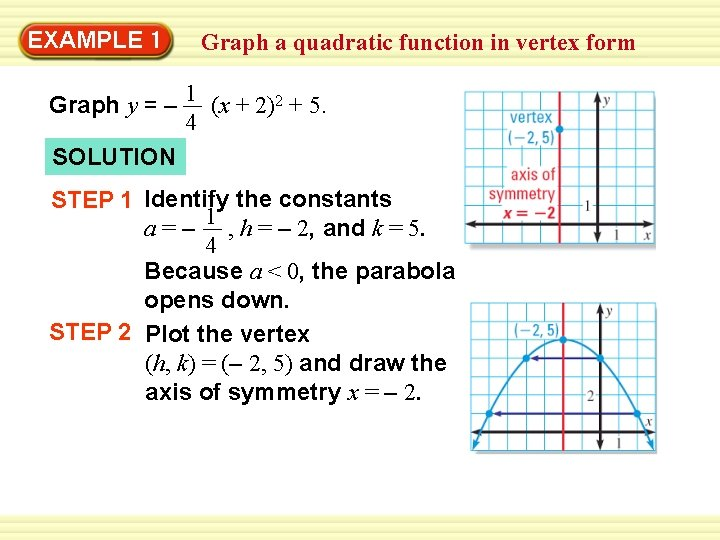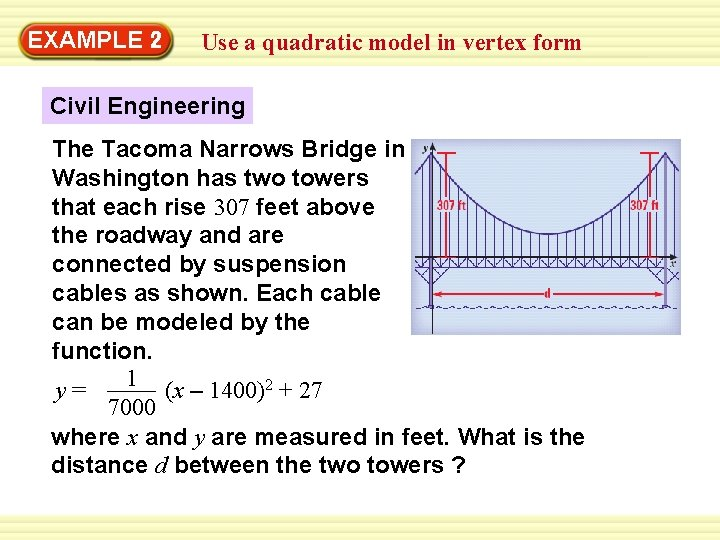# EXAMPLE 1 Graph a quadratic function in vertex

• Slides: 7EXAMPLE 1 Graph a quadratic function in vertex form Graph y = – 1 (x + 2)2 + 5. 4 SOLUTION STEP 1 Identify the constants a = – 1 , h = – 2, and k = 5. 4 Because a < 0, the parabola opens down. STEP 2 Plot the vertex (h, k) = (– 2, 5) and draw the axis of symmetry x = – 2.EXAMPLE 1 Graph a quadratic function in vertex form STEP 3 Evaluate the function for two values of x. 1 – x = 0: y = (0 + 2)2 + 5 = 4 4 1 – x = 2: y = (2 + 2)2 + 5 = 1 4 Plot the points (0, 4) and (2, 1) and their reflections in the axis of symmetry. STEP 4 Draw a parabola through the plotted points.EXAMPLE 2 Use a quadratic model in vertex form Civil Engineering The Tacoma Narrows Bridge in Washington has two towers that each rise 307 feet above the roadway and are connected by suspension cables as shown. Each cable can be modeled by the function. 1 (x – 1400)2 + 27 y= 7000 where x and y are measured in feet. What is the distance d between the two towers ?EXAMPLE 2 Use a quadratic model in vertex form SOLUTION The vertex of the parabola is (1400, 27). So, a cable’s lowest point is 1400 feet from the left tower shown above. Because the heights of the two towers are the same, the symmetry of the parabola implies that the vertex is also 1400 feet from the right tower. So, the distance between the two towers is d = 2 (1400) = 2800 feet.GUIDED PRACTICE for Examples 1 and 2 Graph the function. Label the vertex and axis of symmetry. 1. y = (x + 2)2 – 3 2. y = –(x + 1)2 + 5GUIDED PRACTICE 3. f(x) = 1 (x – 3)2 – 4 2 for Examples 1 and 2GUIDED PRACTICE for Examples 1 and 2 4. WHAT IF? Suppose an architect designs a bridge 1 (x – 1400)2 + 27 with cables that can be modeled by y = 6500 where x and y are measured in feet. Compare this function’s graph to the graph of the function in Example 2. ANSWER This graph is slightly steeper than the graph in Example 2. They both have the same vertex and axis of symmetry, and both open up.